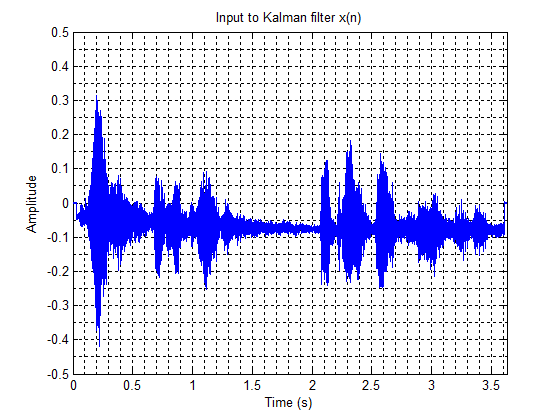# Implementation of the LMS Algorithm

 Page 3/4 Date 31.03.2018 Size 219.46 Kb.
1   2   3   4

#### 2.2.2. Implementation of the LMS Algorithm

LMS algorithm contains iterations, each of which has 3 different steps. These steps are outlined below17.

1. The output of the FIR filter, y(n) is calculated: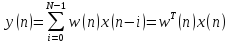1. The value of error is computed: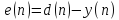1. The tap weights of the FIR vector are updated and prepared for the next iteration: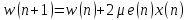As it was previously pointed out, due to the computational simplicity of the LMS algorithm, it is known to be one of the most popular adaptive algorithms. It is important to note that 2N addition, 2N+1 multiplication (N for calculating the output y(n) and one for 2μe(n) and additional N for the scalar by vector multiplication) are required for each iteration of the LMS algorithm18.

3. LMS ALGORITHM COMBINED WITH KALMAN FILTER FOR ECHO CANCELLATION
As it was mentioned in the earlier section, Kalman Filter is regarded as “a set of mathematical equations that provide an efficient computational (recursive) means to estimate the state of a process, in a way that it minimises the mean of the squared error” 7. This means that the minimised squared error will cause the filter output to be closer to the desired signal.

In order to take advantage of this desirable behaviour of the Kalman filter; for the first time, it has been combined with the simplest and most commonly used algorithm of adaptive filter (LMS algorithm) to create a hybrid filter, in an attempt to reduce the effect of the echo in communication channel. By using KF, the minimised squared error will cause the filter output to be closer to the desired signal. Figure 4 depicts the flow diagram of how the code for the combination of LMS and KF in echo cancellation operates.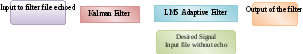Figure 4. Process of KF combined with LMS adaptive filter algorithm
As shown in Figure 4, initially an echoed file is used as an input to the Kalman filter; the signal of this echoed input is depicted in figure 5.